# Selina Solutions Concise Mathematics Class 6 Chapter 14: Fractions Exercise 14(B)

Selina Solutions Concise Mathematics Class 6 Chapter 14: Fractions Exercise 14(B) discusses the concepts such as reducing the fractions to their lowest term, determining the greater and smaller fractions, arranging the fractions in ascending and descending order. The solutions PDF, created by our expert faculty team, helps students to ace the exam confidently. Practising these Solutions on a daily basis, helps them to enhance conceptual knowledge about the concept. Students can refer and download the Selina Solutions Concise Mathematics Class 6 Chapter 14 Fractions Exercise 14(B) PDF links, which are given below.

## Selina Solutions Concise Mathematics Class 6 Chapter 14 Fractions Exercise 14(B) Download PDF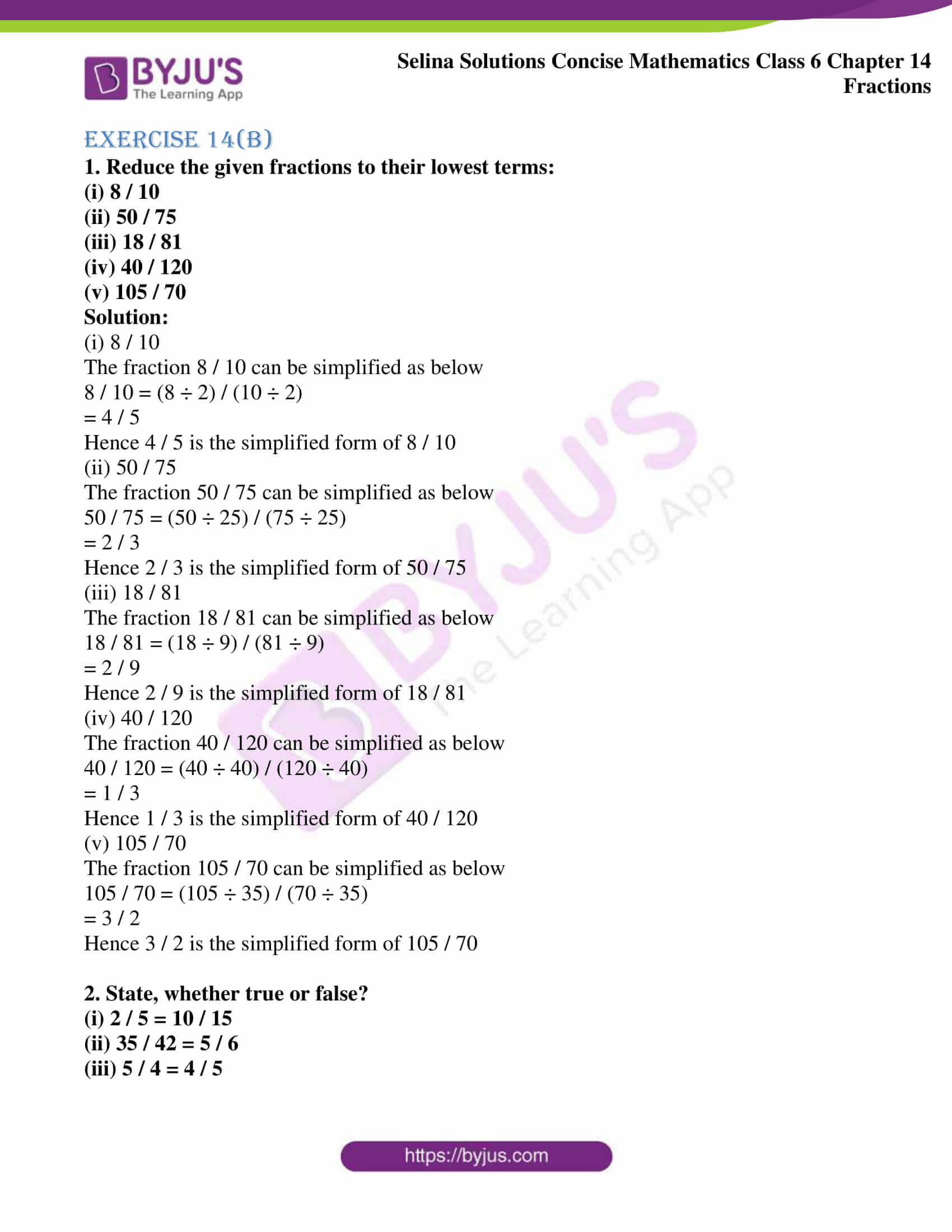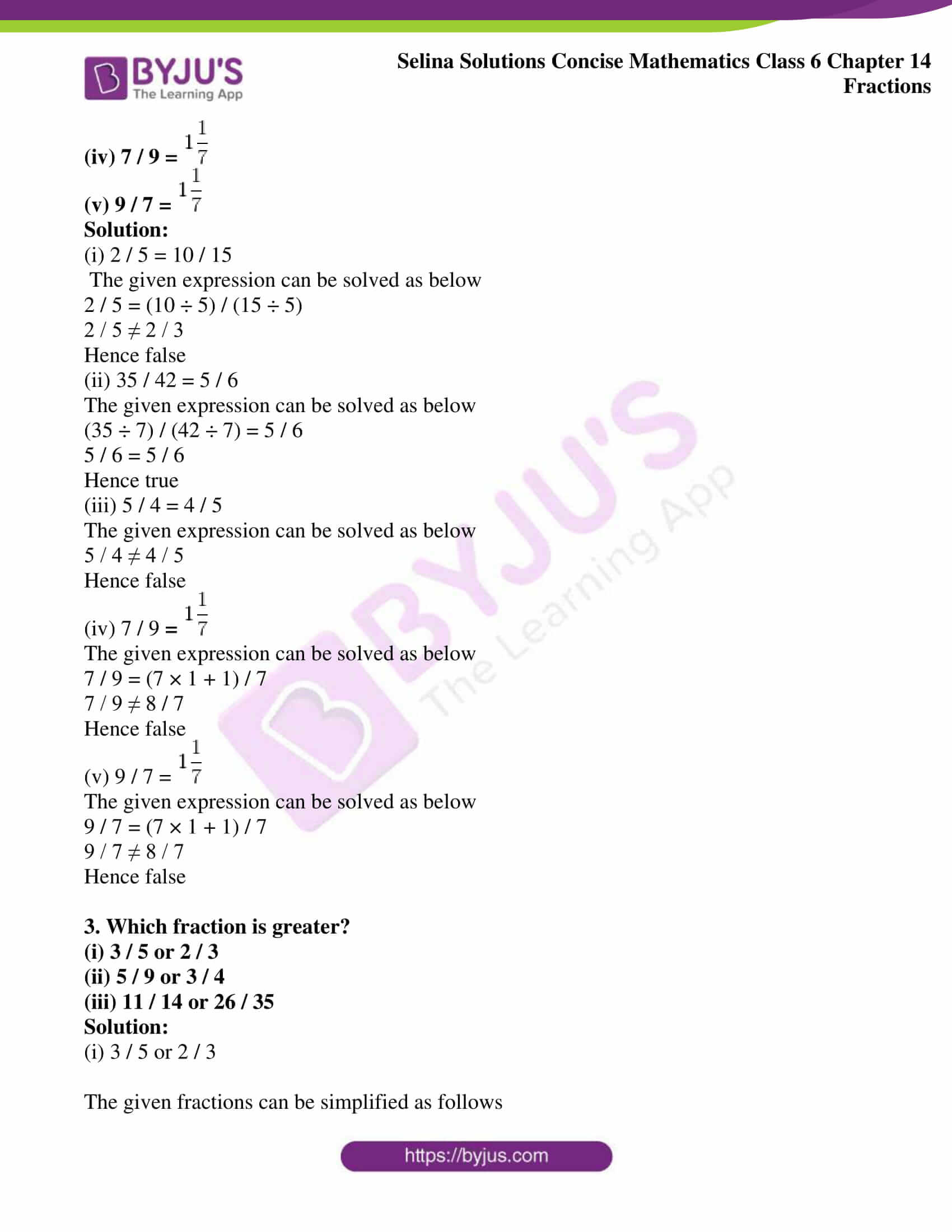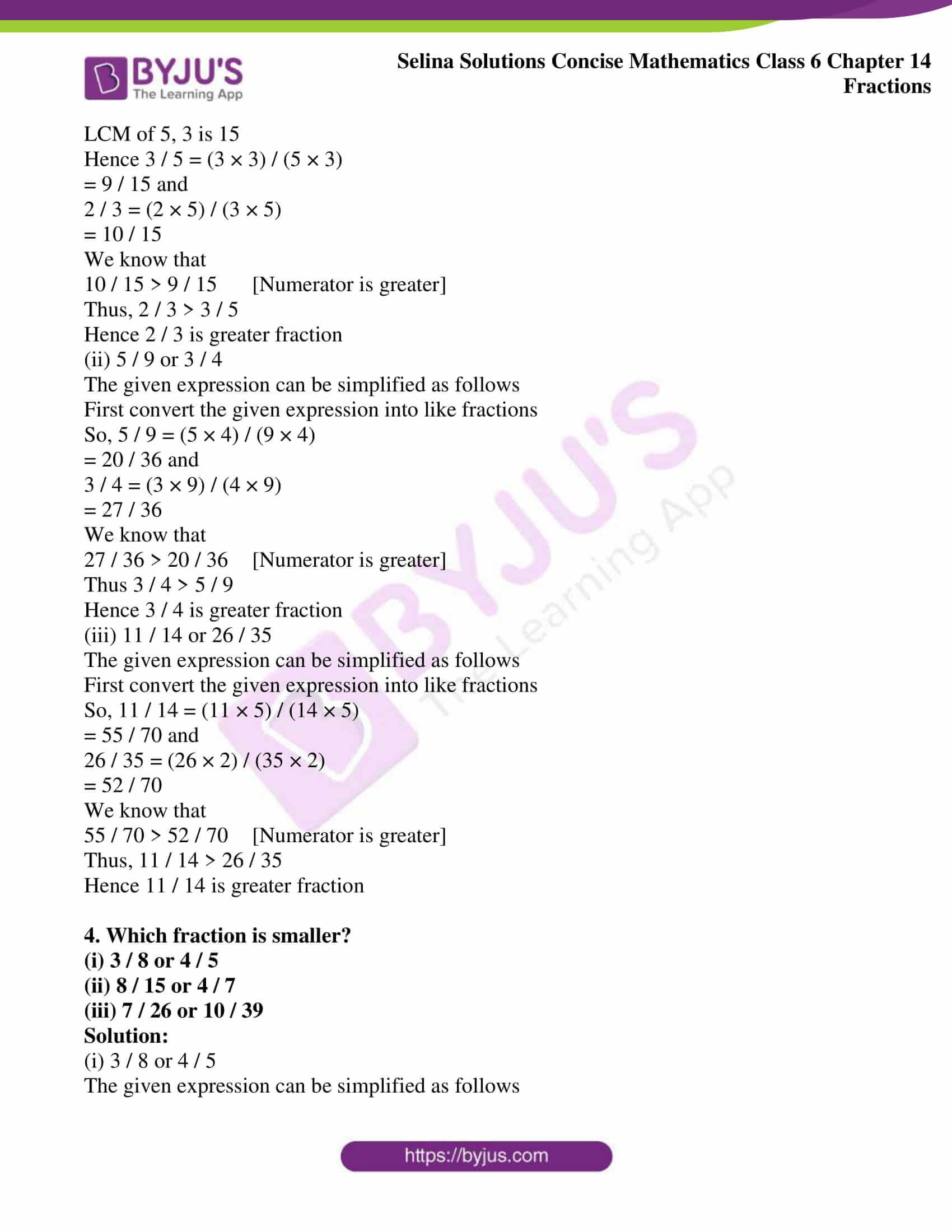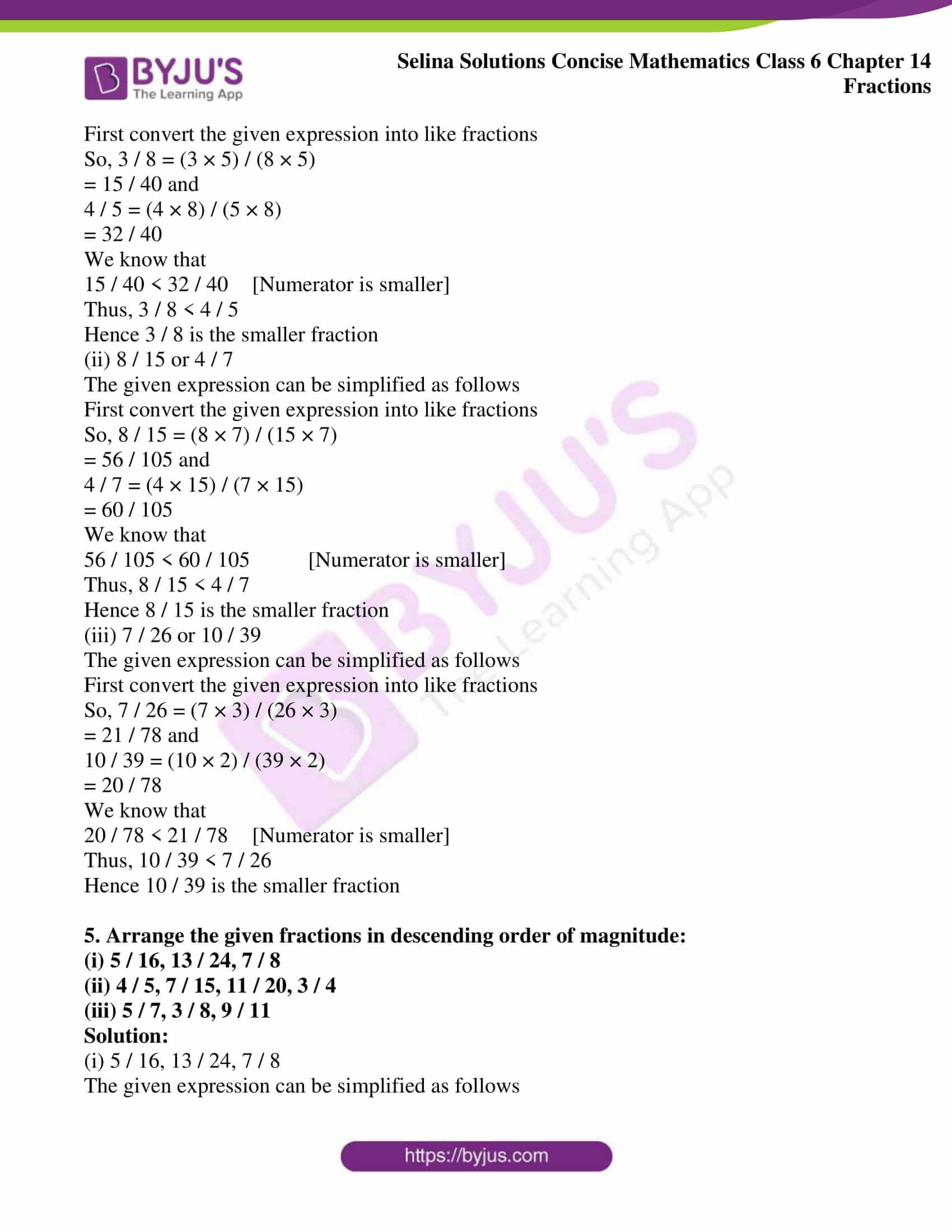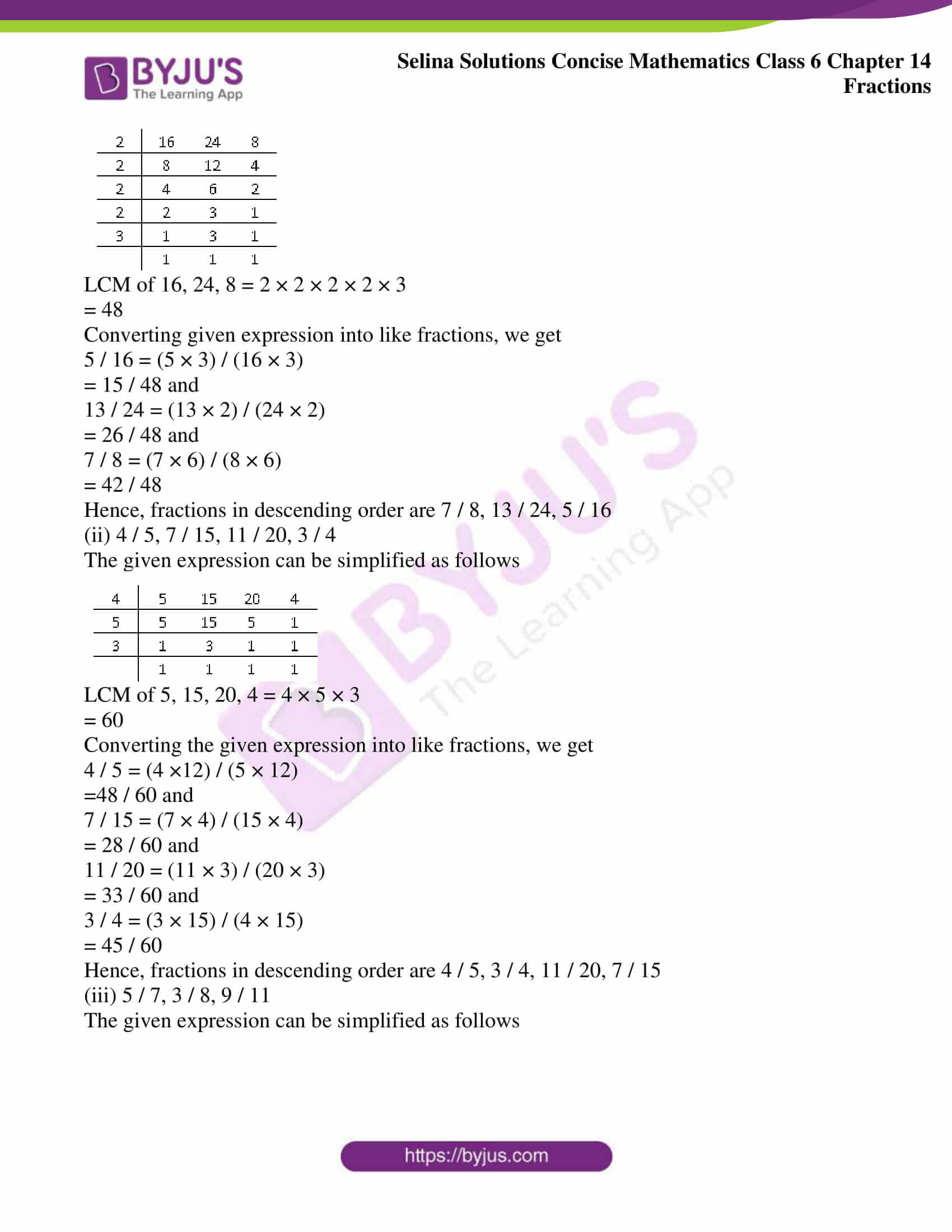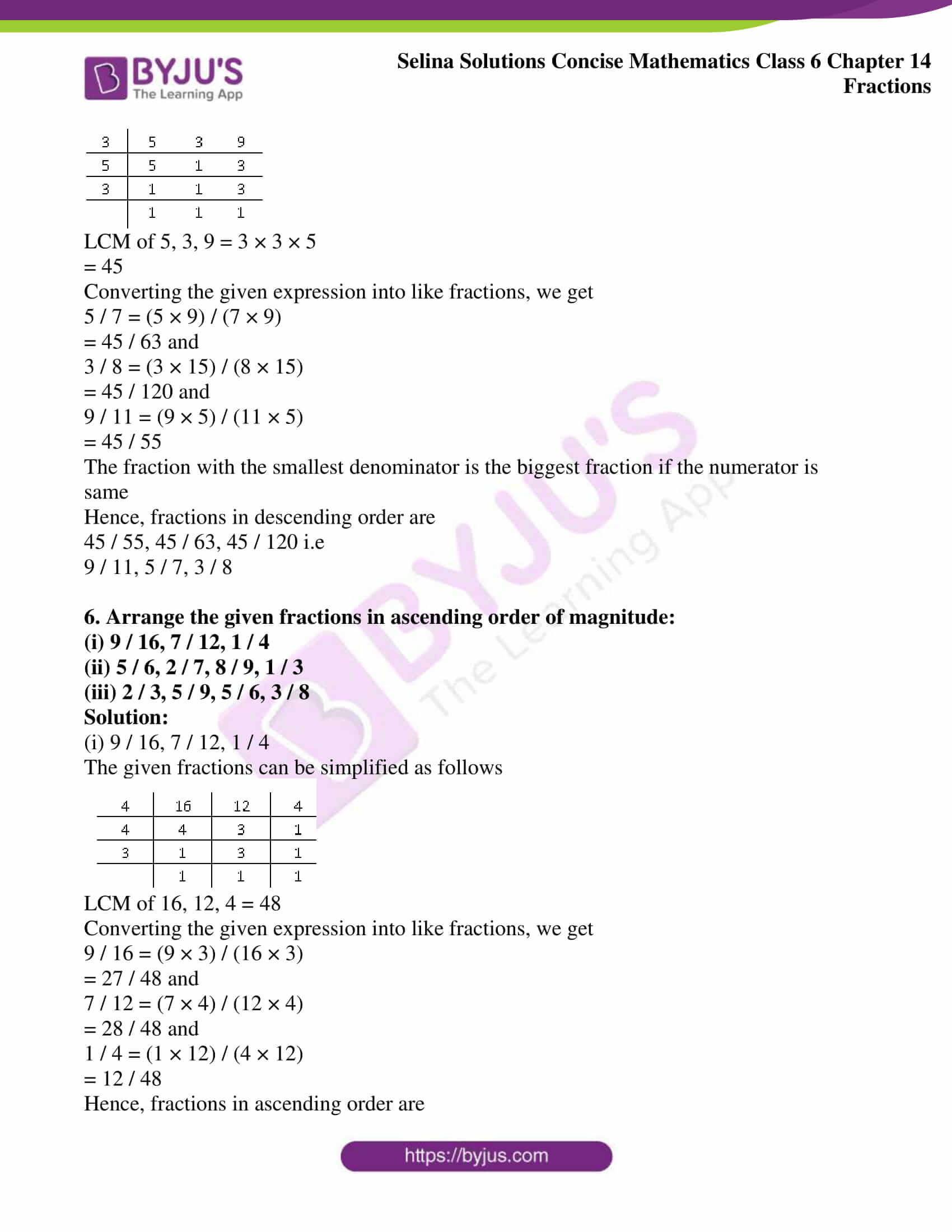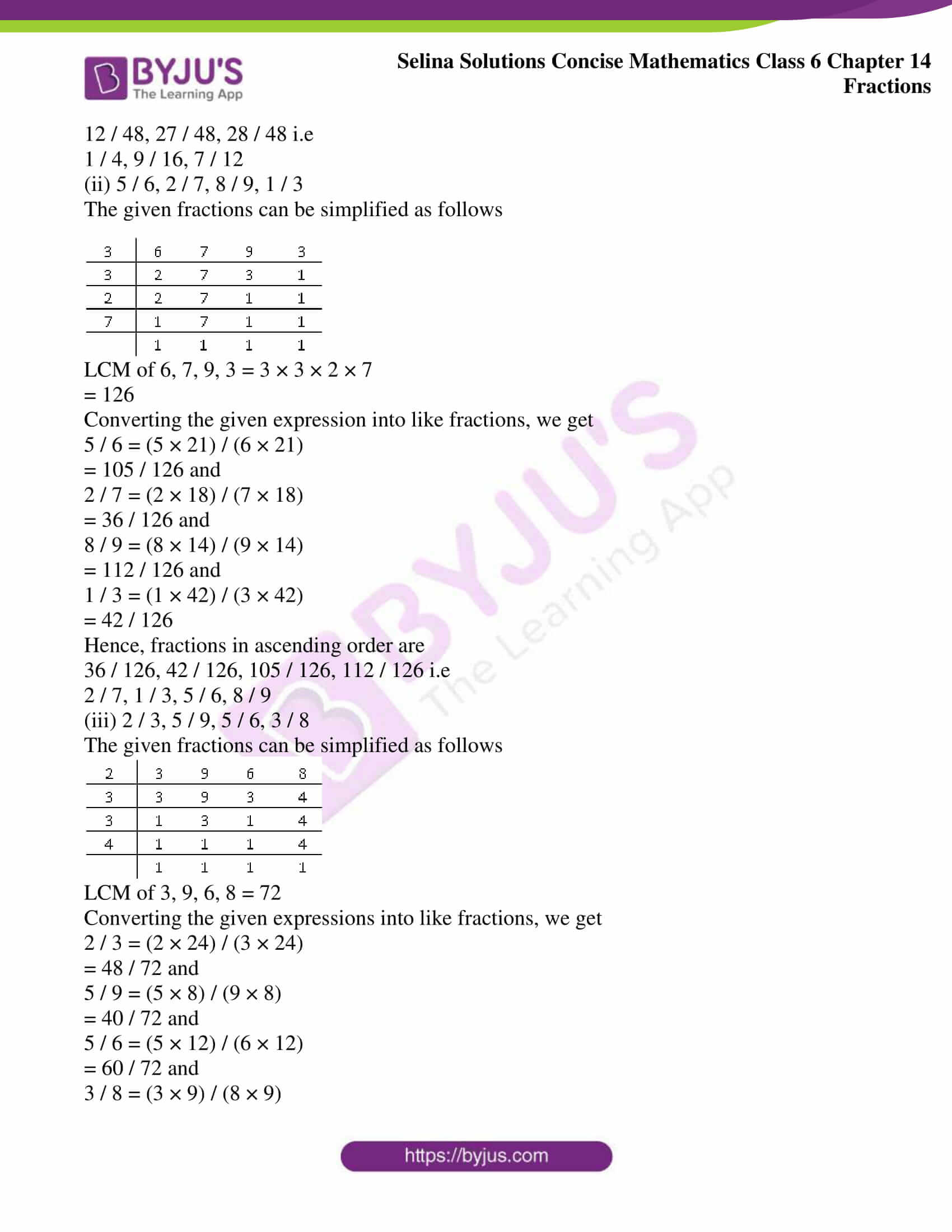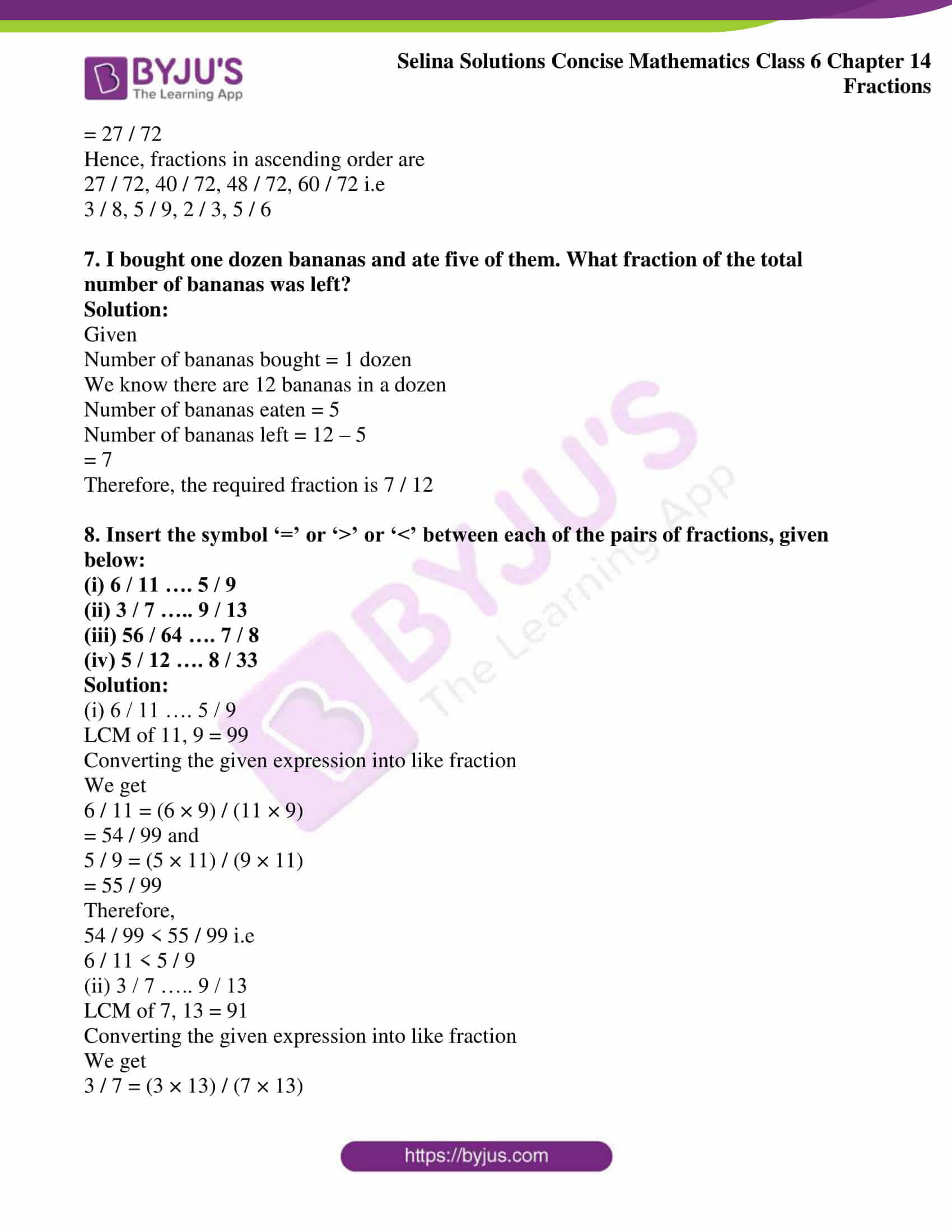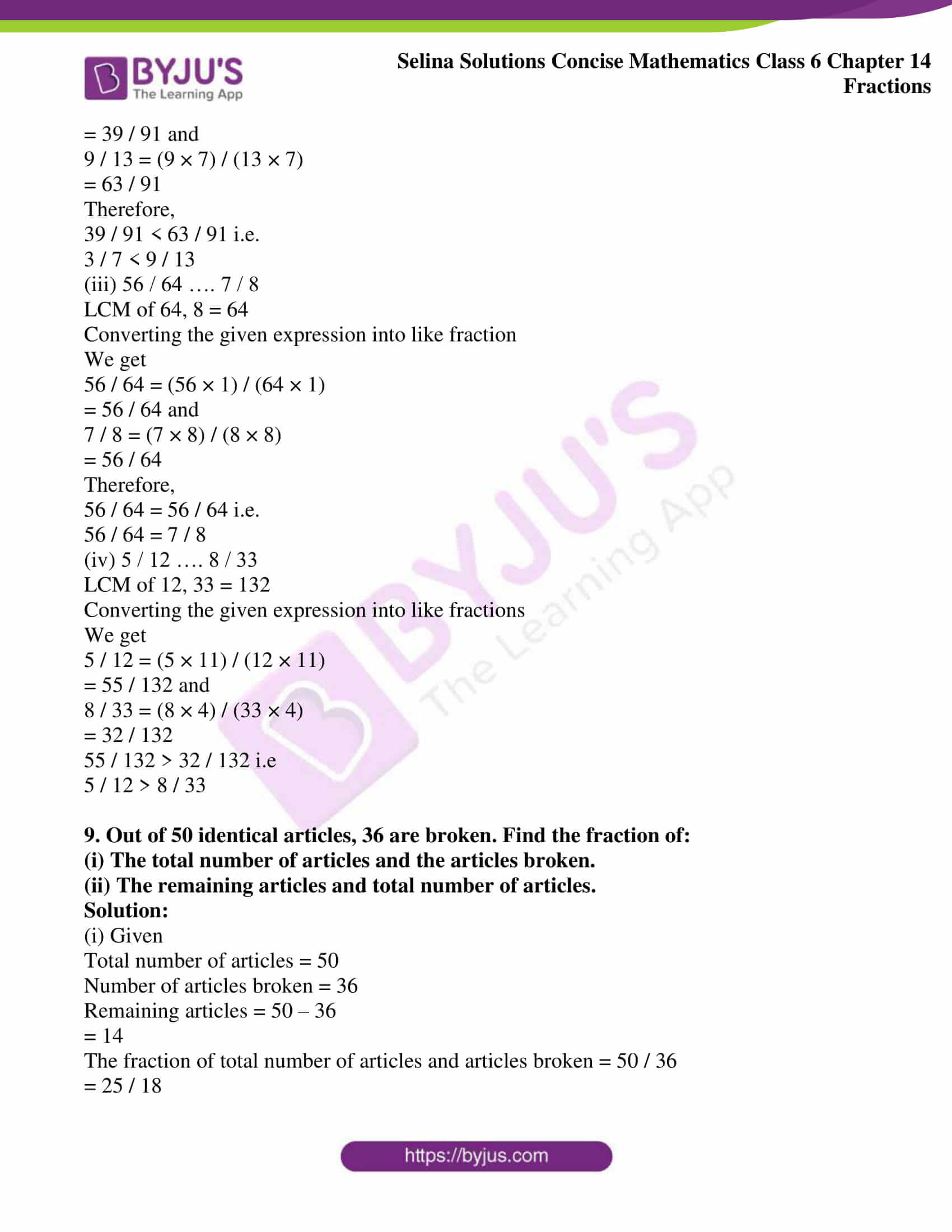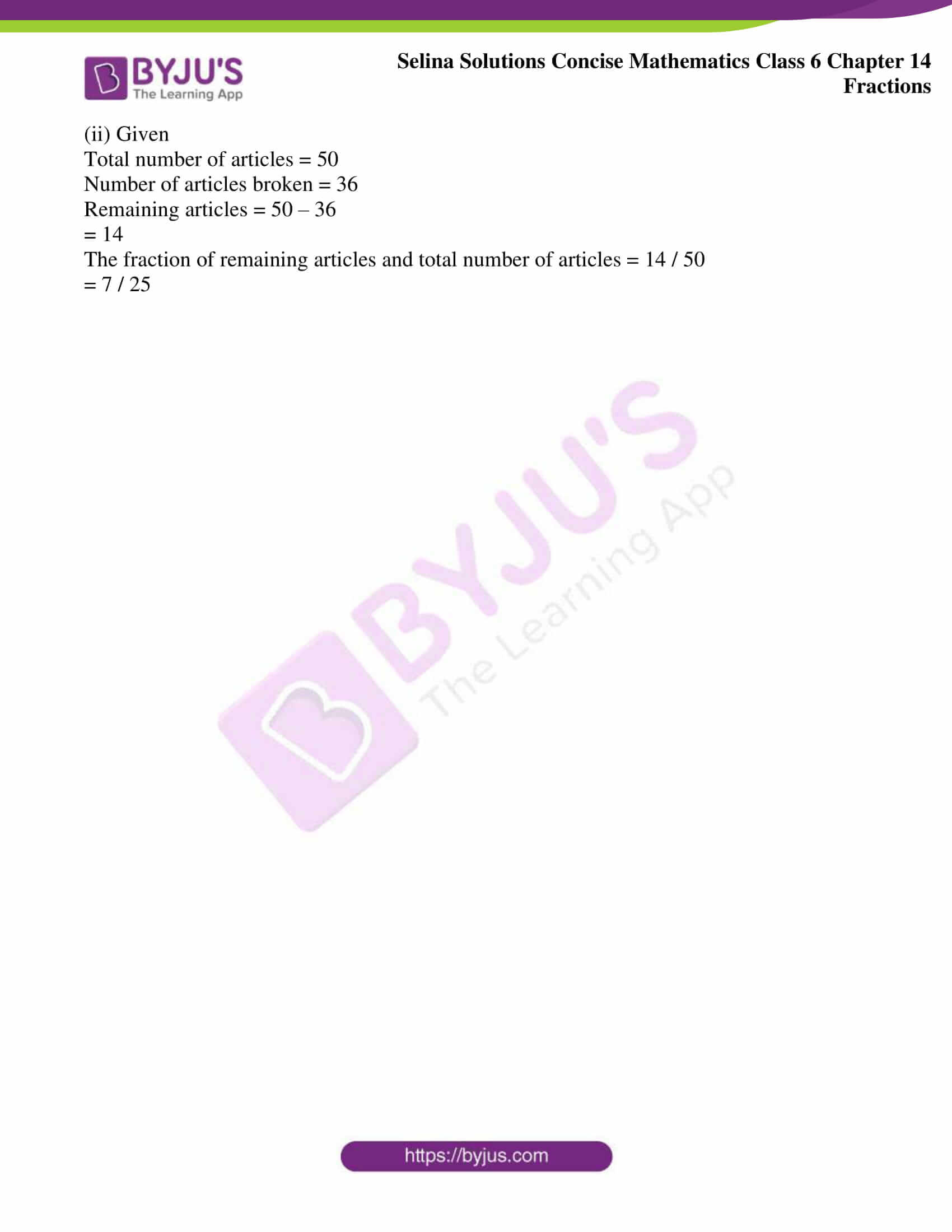### Access other exercises of Selina Solutions Concise Mathematics Class 6 Chapter 14: Fractions

Exercise 14(A) Solutions

Exercise 14(C) Solutions

Exercise 14(D) Solutions

Exercise 14(E) Solutions

### Access Selina Solutions Concise Mathematics Class 6 Chapter 14: Fractions Exercise 14(B)

Exercise 14(B)

1. Reduce the given fractions to their lowest terms:

(i) 8 / 10

(ii) 50 / 75

(iii) 18 / 81

(iv) 40 / 120

(v) 105 / 70

Solution:

(i) 8 / 10

The fraction 8 / 10 can be simplified as below

8 / 10 = (8 ÷ 2) / (10 ÷ 2)

= 4 / 5

Hence 4 / 5 is the simplified form of 8 / 10

(ii) 50 / 75

The fraction 50 / 75 can be simplified as below

50 / 75 = (50 ÷ 25) / (75 ÷ 25)

= 2 / 3

Hence 2 / 3 is the simplified form of 50 / 75

(iii) 18 / 81

The fraction 18 / 81 can be simplified as below

18 / 81 = (18 ÷ 9) / (81 ÷ 9)

= 2 / 9

Hence 2 / 9 is the simplified form of 18 / 81

(iv) 40 / 120

The fraction 40 / 120 can be simplified as below

40 / 120 = (40 ÷ 40) / (120 ÷ 40)

= 1 / 3

Hence 1 / 3 is the simplified form of 40 / 120

(v) 105 / 70

The fraction 105 / 70 can be simplified as below

105 / 70 = (105 ÷ 35) / (70 ÷ 35)

= 3 / 2

Hence 3 / 2 is the simplified form of 105 / 70

2. State, whether true or false?

(i) 2 / 5 = 10 / 15

(ii) 35 / 42 = 5 / 6

(iii) 5 / 4 = 4 / 5

(iv) 7 / 9 =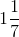(v) 9 / 7 =Solution:

(i) 2 / 5 = 10 / 15

The given expression can be solved as below

2 / 5 = (10 ÷ 5) / (15 ÷ 5)

2 / 5 ≠ 2 / 3

Hence false

(ii) 35 / 42 = 5 / 6

The given expression can be solved as below

(35 ÷ 7) / (42 ÷ 7) = 5 / 6

5 / 6 = 5 / 6

Hence true

(iii) 5 / 4 = 4 / 5

The given expression can be solved as below

5 / 4 ≠ 4 / 5

Hence false

(iv) 7 / 9 =The given expression can be solved as below

7 / 9 = (7 × 1 + 1) / 7

7 / 9 ≠ 8 / 7

Hence false

(v) 9 / 7 =The given expression can be solved as below

9 / 7 = (7 × 1 + 1) / 7

9 / 7 ≠ 8 / 7

Hence false

3. Which fraction is greater?

(i) 3 / 5 or 2 / 3

(ii) 5 / 9 or 3 / 4

(iii) 11 / 14 or 26 / 35

Solution:

(i) 3 / 5 or 2 / 3

The given fractions can be simplified as follows

LCM of 5, 3 is 15

Hence 3 / 5 = (3 × 3) / (5 × 3)

= 9 / 15 and

2 / 3 = (2 × 5) / (3 × 5)

= 10 / 15

We know that

10 / 15 > 9 / 15 [Numerator is greater]

Thus, 2 / 3 > 3 / 5

Hence 2 / 3 is greater fraction

(ii) 5 / 9 or 3 / 4

The given expression can be simplified as follows

First convert the given expression into like fractions

So, 5 / 9 = (5 × 4) / (9 × 4)

= 20 / 36 and

3 / 4 = (3 × 9) / (4 × 9)

= 27 / 36

We know that

27 / 36 > 20 / 36 [Numerator is greater]

Thus 3 / 4 > 5 / 9

Hence 3 / 4 is greater fraction

(iii) 11 / 14 or 26 / 35

The given expression can be simplified as follows

First convert the given expression into like fractions

So, 11 / 14 = (11 × 5) / (14 × 5)

= 55 / 70 and

26 / 35 = (26 × 2) / (35 × 2)

= 52 / 70

We know that

55 / 70 > 52 / 70 [Numerator is greater]

Thus, 11 / 14 > 26 / 35

Hence 11 / 14 is greater fraction

4. Which fraction is smaller?

(i) 3 / 8 or 4 / 5

(ii) 8 / 15 or 4 / 7

(iii) 7 / 26 or 10 / 39

Solution:

(i) 3 / 8 or 4 / 5

The given expression can be simplified as follows

First convert the given expression into like fractions

So, 3 / 8 = (3 × 5) / (8 × 5)

= 15 / 40 and

4 / 5 = (4 × 8) / (5 × 8)

= 32 / 40

We know that

15 / 40 < 32 / 40 [Numerator is smaller]

Thus, 3 / 8 < 4 / 5

Hence 3 / 8 is the smaller fraction

(ii) 8 / 15 or 4 / 7

The given expression can be simplified as follows

First convert the given expression into like fractions

So, 8 / 15 = (8 × 7) / (15 × 7)

= 56 / 105 and

4 / 7 = (4 × 15) / (7 × 15)

= 60 / 105

We know that

56 / 105 < 60 / 105 [Numerator is smaller]

Thus, 8 / 15 < 4 / 7

Hence 8 / 15 is the smaller fraction

(iii) 7 / 26 or 10 / 39

The given expression can be simplified as follows

First convert the given expression into like fractions

So, 7 / 26 = (7 × 3) / (26 × 3)

= 21 / 78 and

10 / 39 = (10 × 2) / (39 × 2)

= 20 / 78

We know that

20 / 78 < 21 / 78 [Numerator is smaller]

Thus, 10 / 39 < 7 / 26

Hence 10 / 39 is the smaller fraction

5. Arrange the given fractions in descending order of magnitude:

(i) 5 / 16, 13 / 24, 7 / 8

(ii) 4 / 5, 7 / 15, 11 / 20, 3 / 4

(iii) 5 / 7, 3 / 8, 9 / 11

Solution:

(i) 5 / 16, 13 / 24, 7 / 8

The given expression can be simplified as follows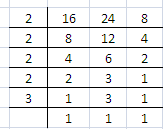LCM of 16, 24, 8 = 2 × 2 × 2 × 2 × 3

= 48

Converting given expression into like fractions, we get

5 / 16 = (5 × 3) / (16 × 3)

= 15 / 48 and

13 / 24 = (13 × 2) / (24 × 2)

= 26 / 48 and

7 / 8 = (7 × 6) / (8 × 6)

= 42 / 48

Hence, fractions in descending order are 7 / 8, 13 / 24, 5 / 16

(ii) 4 / 5, 7 / 15, 11 / 20, 3 / 4

The given expression can be simplified as follows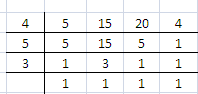LCM of 5, 15, 20, 4 = 4 × 5 × 3

= 60

Converting the given expression into like fractions, we get

4 / 5 = (4 ×12) / (5 × 12)

=48 / 60 and

7 / 15 = (7 × 4) / (15 × 4)

= 28 / 60 and

11 / 20 = (11 × 3) / (20 × 3)

= 33 / 60 and

3 / 4 = (3 × 15) / (4 × 15)

= 45 / 60

Hence, fractions in descending order are 4 / 5, 3 / 4, 11 / 20, 7 / 15

(iii) 5 / 7, 3 / 8, 9 / 11

The given expression can be simplified as follows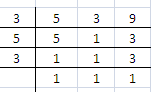LCM of 5, 3, 9 = 3 × 3 × 5

= 45

Converting the given expression into like fractions, we get

5 / 7 = (5 × 9) / (7 × 9)

= 45 / 63 and

3 / 8 = (3 × 15) / (8 × 15)

= 45 / 120 and

9 / 11 = (9 × 5) / (11 × 5)

= 45 / 55

The fraction with the smallest denominator is the biggest fraction if the numerator is same

Hence, fractions in descending order are

45 / 55, 45 / 63, 45 / 120 i.e

9 / 11, 5 / 7, 3 / 8

6. Arrange the given fractions in ascending order of magnitude:

(i) 9 / 16, 7 / 12, 1 / 4

(ii) 5 / 6, 2 / 7, 8 / 9, 1 / 3

(iii) 2 / 3, 5 / 9, 5 / 6, 3 / 8

Solution:

(i) 9 / 16, 7 / 12, 1 / 4

The given fractions can be simplified as follows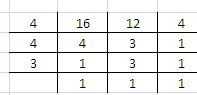LCM of 16, 12, 4 = 48

Converting the given expression into like fractions, we get

9 / 16 = (9 × 3) / (16 × 3)

= 27 / 48 and

7 / 12 = (7 × 4) / (12 × 4)

= 28 / 48 and

1 / 4 = (1 × 12) / (4 × 12)

= 12 / 48

Hence, fractions in ascending order are

12 / 48, 27 / 48, 28 / 48 i.e

1 / 4, 9 / 16, 7 / 12

(ii) 5 / 6, 2 / 7, 8 / 9, 1 / 3

The given fractions can be simplified as follows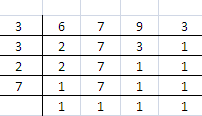LCM of 6, 7, 9, 3 = 3 × 3 × 2 × 7

= 126

Converting the given expression into like fractions, we get

5 / 6 = (5 × 21) / (6 × 21)

= 105 / 126 and

2 / 7 = (2 × 18) / (7 × 18)

= 36 / 126 and

8 / 9 = (8 × 14) / (9 × 14)

= 112 / 126 and

1 / 3 = (1 × 42) / (3 × 42)

= 42 / 126

Hence, fractions in ascending order are

36 / 126, 42 / 126, 105 / 126, 112 / 126 i.e

2 / 7, 1 / 3, 5 / 6, 8 / 9

(iii) 2 / 3, 5 / 9, 5 / 6, 3 / 8

The given fractions can be simplified as follows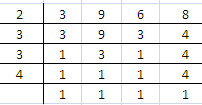LCM of 3, 9, 6, 8 = 72

Converting the given expressions into like fractions, we get

2 / 3 = (2 × 24) / (3 × 24)

= 48 / 72 and

5 / 9 = (5 × 8) / (9 × 8)

= 40 / 72 and

5 / 6 = (5 × 12) / (6 × 12)

= 60 / 72 and

3 / 8 = (3 × 9) / (8 × 9)

= 27 / 72

Hence, fractions in ascending order are

27 / 72, 40 / 72, 48 / 72, 60 / 72 i.e

3 / 8, 5 / 9, 2 / 3, 5 / 6

7. I bought one dozen bananas and ate five of them. What fraction of the total number of bananas was left?

Solution:

Given

Number of bananas bought = 1 dozen

We know there are 12 bananas in a dozen

Number of bananas eaten = 5

Number of bananas left = 12 – 5

= 7

Therefore, the required fraction is 7 / 12

8. Insert the symbol ‘=’ or ‘>’ or ‘<’ between each of the pairs of fractions, given below:

(i) 6 / 11 …. 5 / 9

(ii) 3 / 7 ….. 9 / 13

(iii) 56 / 64 …. 7 / 8

(iv) 5 / 12 …. 8 / 33

Solution:

(i) 6 / 11 …. 5 / 9

LCM of 11, 9 = 99

Converting the given expression into like fraction

We get

6 / 11 = (6 × 9) / (11 × 9)

= 54 / 99 and

5 / 9 = (5 × 11) / (9 × 11)

= 55 / 99

Therefore,

54 / 99 < 55 / 99 i.e

6 / 11 < 5 / 9

(ii) 3 / 7 ….. 9 / 13

LCM of 7, 13 = 91

Converting the given expression into like fraction

We get

3 / 7 = (3 × 13) / (7 × 13)

= 39 / 91 and

9 / 13 = (9 × 7) / (13 × 7)

= 63 / 91

Therefore,

39 / 91 < 63 / 91 i.e.

3 / 7 < 9 / 13

(iii) 56 / 64 …. 7 / 8

LCM of 64, 8 = 64

Converting the given expression into like fraction

We get

56 / 64 = (56 × 1) / (64 × 1)

= 56 / 64 and

7 / 8 = (7 × 8) / (8 × 8)

= 56 / 64

Therefore,

56 / 64 = 56 / 64 i.e.

56 / 64 = 7 / 8

(iv) 5 / 12 …. 8 / 33

LCM of 12, 33 = 132

Converting the given expression into like fractions

We get

5 / 12 = (5 × 11) / (12 × 11)

= 55 / 132 and

8 / 33 = (8 × 4) / (33 × 4)

= 32 / 132

55 / 132 > 32 / 132 i.e

5 / 12 > 8 / 33

9. Out of 50 identical articles, 36 are broken. Find the fraction of:

(i) The total number of articles and the articles broken.

(ii) The remaining articles and total number of articles.

Solution:

(i) Given

Total number of articles = 50

Number of articles broken = 36

Remaining articles = 50 – 36

= 14

The fraction of total number of articles and articles broken = 50 / 36

= 25 / 18

(ii) Given

Total number of articles = 50

Number of articles broken = 36

Remaining articles = 50 – 36

= 14

The fraction of remaining articles and total number of articles = 14 / 50

= 7 / 25